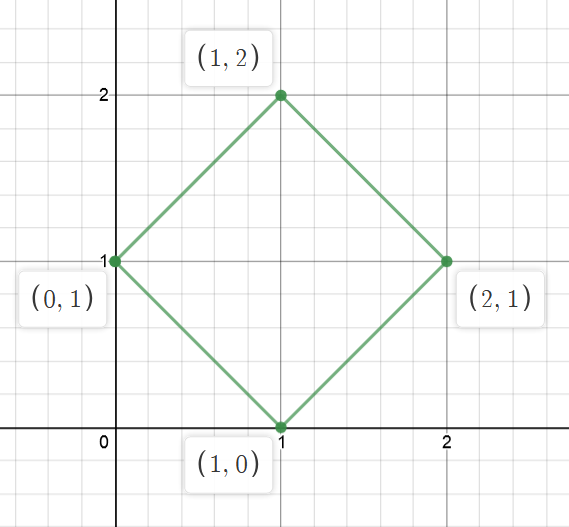Check Square

Posted: 31 Dec, 2020
Difficulty: Moderate

PROBLEM STATEMENT

Can you check if those four points make a square?

Example:
Let the input be [1,0,2,1] and [0,1,1,2].
So, the coordinates of the four points be [ {1, 0}, {0, 1}, {2, 1}, {1, 2} ]From the above image, we can see that it is a square. Thus, the output will be ‘Yes’.
Input format:
The first line of input contains an integer ‘T’ denoting the number of test cases.

The first line of each test case contains four space-separated integers representing x-coordinates of the four points.

The second line of each test case contains four space-separated integers representing y-coordinates of the four points.
Output format :
For each test case, print ‘Yes’ if four points make a square otherwise print ‘No’.
Note:
Don’t print anything, just return True if four points make a square otherwise return False.
Constraints:
1 <= T <= 10^4
-10^9 <= xi, yi <= 10^9

Time limit: 1 secApproach 1

The simple approach would be checking the distance between each pair of points and confirming whether it is a square or not.

• Calculate the square of distance between each pair of points. We are using square of distance to maintain precision.
• Thus, we have 6 values of square of distance between each pair of points as we have 4 points.
• Now check the following:
• Four of the values are equal to each other representing the side of the square.
• The remaining two values are equal to each other representing the diagonal of the square.
• The two equal values are twice of four equal values representing the relationship between side and the diagonal as here the values are square of distances (2 * side^2 = diagonal^2).
• Each value must be greater than 0.